# Create A Program to Create Beach Mining System in Matlab Assignment Solution.

## Instructions

Objective
Write a program to create beach mining system in matlab language.

## Requirements and Specifications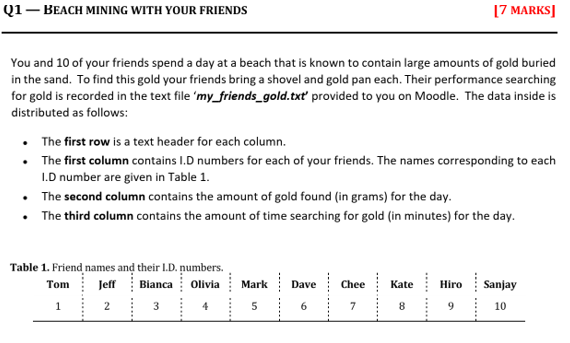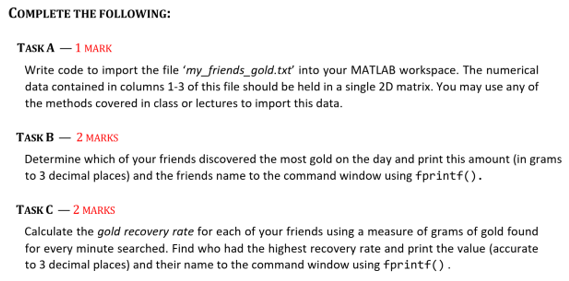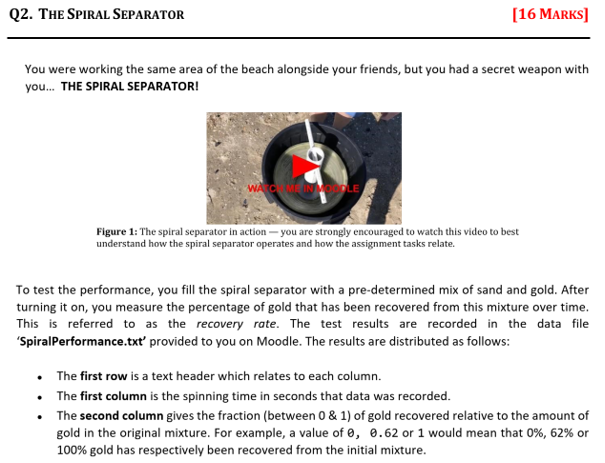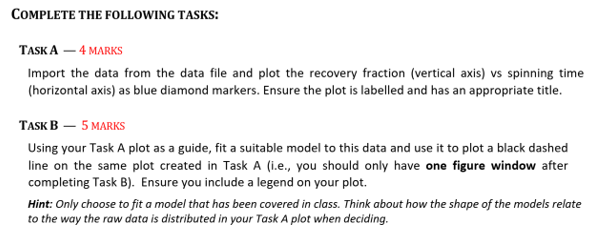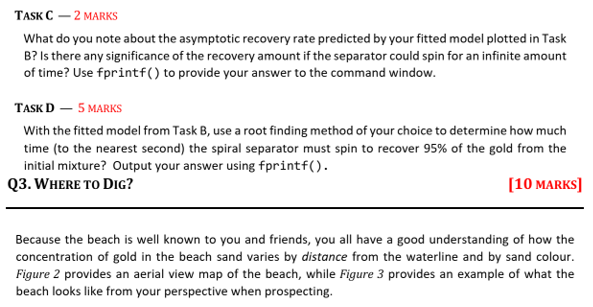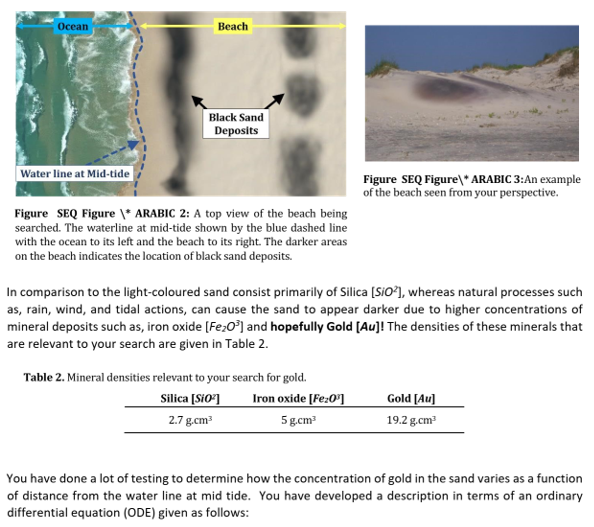Source Code

QUESTION 1

% Q1 % Some code may already be provided below % DO NOT clear, close or clc inside this script % Apply good programming practices % % Name : % ID : % Date Modified : fprintf('\n Q1 \n\n') %% %Add your code here % Define the names for each Friend friend_names = {'Tom', 'Jeff', 'Bianca', 'Olivia', 'Mark', 'Dave', 'Chee', 'Kate', 'Hiro', 'Sanjay'}; %% Task A: Read file my_friends_gold.txt fprintf('\nTask A\n\n'); fid = fopen('my_friends_gold.txt','rt'); M = cell2mat(textscan(fid, '%f%f%f', 'HeaderLines',1)); fclose(fid); disp(M) %% Task B: Find the row with the max amount of gold fprintf('\nTask B\n\n'); [max_gold, row_id] = max(M(:,2)); max_gold_friend_id = M(row_id,1); fprintf("%.3f (g)\n", max_gold); fprintf("%s\n", friend_names{max_gold_friend_id}); %% Task C: Calculate the gold recovery rate for each friend and then find the one with the highest rate fprintf('\nTask C\n\n'); recovery_rate = M(:,2)./M(:,3); % grams / min % Now, get the max recovery rate and the row id [max_recovery, row_id] = max(recovery_rate); max_recovery_friend_id = M(row_id,1); fprintf("%.3f (g/min)\n", max_recovery); fprintf("%s\n", friend_names{max_recovery_friend_id}); %% Task D: Best performance in terms of gold value fprintf('\nTask D\n\n'); price = 80; % gold price per gram % From Task C, we have a vector of recovery rate as grams-per-min for each friend. If we multiply % this vector by the price, we obtain the performance in dollar-per-min dollar_per_min = price*recovery_rate; % Now, to convert these values from dollar-per-min to dollar-per-hour we % have to multiply the values by 60 dollar_per_hour = dollar_per_min*60; % performance % Now, select the friend with the best performance [max_perform, row_id] = max(dollar_per_hour); max_perform_friend_id = M(row_id, 1); % Now print fprintf("%.2f ($/hr)\n", max_perform); fprintf("%s\n", friend_names{max_perform_friend_id}); % Print results  QUESTION 2 % Q2 % Some code may already be provided below % DO NOT clear, close or clc inside this script % Apply good programming practices % % Name : % ID : % Date Modified : fprintf('\n Q2 \n\n') %% %Add your code here %% Task A: Read data fprintf('\nTask A\n\n'); fid = fopen('SpiralPerformance.txt','rt'); M = cell2mat(textscan(fid, '%f%f', 'HeaderLines',1)); fclose(fid); disp(M); % Now plot figure(1) plot(M(:,1), M(:, 2), 'bd') xlabel('Spinning Time (s)'); ylabel('Fraction of gold recovered'); title('Fraction of gold recovered vs. Spinning Time') %% Task B: Fit model fprintf('\nTask B (See Figure 1)\n\n'); % The model looks polynomial so we will fit to an exponential function % y = a*x^b % log(y) = log(a) + b*log(x) % Y = A + B*X % Where % Y = log(y) % A = log(a) % B = b % X = log(x) % Store data in two vectors x = M(:,1); y = M(:,2); Y = log(y); X = log(x); % GEt number of points n = length(x); % Define the matrix for Least-Squares method A = zeros(n, 2); A(:,1) = 1; A(:,2) = X; % Define vector b b = Y; % Now, calculate coefficients with the following formula % coefficients = inv(A'*A)*A'*b coeffs = inv(A'*A)*A'*b; % Now, calculate a and b a = exp(coeffs(1)); b = coeffs(2); % Now, calculate fitted values y_fit = a*x.^b; % % Plot figure(1) hold on plot(x, y_fit, 'k--') grid on legend('Data', 'Fit') %% Task C fprintf('\nTask C\n\n'); fprintf("As the Spinning Time increases, the fraction of recovered gold also increases, but the rate decreases\nmore and more. If the curve is extended for infinite Spinning Time values, the increase\nin the fraction of recovered gold would decrease to zero, which means that the rate of recovered\ngold would not continue to increase, but would reach its maximum and remain constant at that value.\n"); %% Task D fprintf('\nTask D\n\n'); % First, we define the function handle with the coefficients obtained f = @(x)(a*x.^b-0.95); % We also the fine the first derivative of the function df = @(x)(a*b*x.^(b-1)); % Now, we will use Newton-Raphson method to fin the root % Define tolerance, initial error and seed value eps = 1e-3; x0 = 60; err = Inf; xsol(1) = 30; n = 1; while(err > eps) xsol(n+1) = xsol(n) - f(xsol(n))/df(xsol(n)); % Update error err = abs(xsol(n+1)-xsol(n)); % Increase n n = n + 1; end fprintf("The time the spiral separator must spin to recover 95%% is %d(s)\n", round(xsol(end))); %Print results  QUESTION 3 % Q2 % Some code may already be provided below % DO NOT clear, close or clc inside this script % Apply good programming practices % % Name : % ID : % Date Modified : fprintf('\n Q2 \n\n') %% %Add your code here %% Task A: Read data fprintf('\nTask A\n\n'); fid = fopen('SpiralPerformance.txt','rt'); M = cell2mat(textscan(fid, '%f%f', 'HeaderLines',1)); fclose(fid); disp(M); % Now plot figure(1) plot(M(:,1), M(:, 2), 'bd') xlabel('Spinning Time (s)'); ylabel('Fraction of gold recovered'); title('Fraction of gold recovered vs. Spinning Time') %% Task B: Fit model fprintf('\nTask B (See Figure 1)\n\n'); % The model looks polynomial so we will fit to an exponential function % y = a*x^b % log(y) = log(a) + b*log(x) % Y = A + B*X % Where % Y = log(y) % A = log(a) % B = b % X = log(x) % Store data in two vectors x = M(:,1); y = M(:,2); Y = log(y); X = log(x); % GEt number of points n = length(x); % Define the matrix for Least-Squares method A = zeros(n, 2); A(:,1) = 1; A(:,2) = X; % Define vector b b = Y; % Now, calculate coefficients with the following formula % coefficients = inv(A'*A)*A'*b coeffs = inv(A'*A)*A'*b; % Now, calculate a and b a = exp(coeffs(1)); b = coeffs(2); % Now, calculate fitted values y_fit = a*x.^b; % % Plot figure(1) hold on plot(x, y_fit, 'k--') grid on legend('Data', 'Fit') %% Task C fprintf('\nTask C\n\n'); fprintf("As the Spinning Time increases, the fraction of recovered gold also increases, but the rate decreases\nmore and more. If the curve is extended for infinite Spinning Time values, the increase\nin the fraction of recovered gold would decrease to zero, which means that the rate of recovered\ngold would not continue to increase, but would reach its maximum and remain constant at that value.\n"); %% Task D fprintf('\nTask D\n\n'); % First, we define the function handle with the coefficients obtained f = @(x)(a*x.^b-0.95); % We also the fine the first derivative of the function df = @(x)(a*b*x.^(b-1)); % Now, we will use Newton-Raphson method to fin the root % Define tolerance, initial error and seed value eps = 1e-3; x0 = 60; err = Inf; xsol(1) = 30; n = 1; while(err > eps) xsol(n+1) = xsol(n) - f(xsol(n))/df(xsol(n)); % Update error err = abs(xsol(n+1)-xsol(n)); % Increase n n = n + 1; end fprintf("The time the spiral separator must spin to recover 95%% is %d(s)\n", round(xsol(end))); %Print results  QUESTION 4 % Q4 % Some code may already be provided below % DO NOT clear, close or clc inside this script % Apply good programming practices % % Name : % ID : % Date Modified : fprintf('\n Q4 \n\n') %% %Add your code here %% Task A: fprintf('\nTask A\n\n'); % Define the total time of simulation, shovel capacity, time to dig a % shovel, etc T = 5*60*60; % simulation time in seconds dt = 1;% step in seconds shov_l = 3; LITERS_PER_SHOVEL = 5/3; shov_t = 10; CLEANING_TRIGGER_LITERS = 15; % every 15 liters a cleaning process is required clean_t = 120; SHOVEL_WIDTH = 20; % IN CM % calculate the volume of dirt in the shovel V = (SHOVEL_WIDTH/100)^3; % For the Separator Performance, we will use the equation fitted in Q2 performance = @(x)(a*x^b); % We assume we dig in the point of max. concentration of gold, found in Q3 c_max_loc = c_max_dist; % Pick the best SPINNING_TIME spinning_times = 0:1:120; m = zeros(1, length(spinning_times)); for i = 1:length(spinning_times) % Define variables to track the number of digging, the amount of gold % recovered, number of cleanings, etc n_cleanings = 0; n_digs = 0; % Define the spinning time SPINNING_TIME = spinning_times(i); % m = 0; % amount of gold recovered t = 0; liters = 0; while t < T if liters > 0 && mod(liters, CLEANING_TRIGGER_LITERS) == 0 % a cleaning is necessary t = t + clean_t; n_cleanings = n_cleanings + 1; liters = 0; else for j = 1:3 % Dig one load t = t + shov_l*shov_t; % time for 3 shovels % Recover gold m(i) = m(i) + performance(SPINNING_TIME)*V*c_max; t = t + SPINNING_TIME; n_digs = n_digs + 1; liters = liters + 5; end end end end fprintf("For our formula, we will use the following variables\n\n"); fprintf("\tx: Spinning time (s)\n\n"); fprintf("\tT: total time of simulation (5 hours)\n\n"); fprintf("\tshov_t: Time that takes to dig the shovel and extract dirt\n\n"); fprintf("\tshov_l: Number of shovel loads required to process a load in the spiral separator\n\n"); fprintf("\tclean_t: Time that takes to clean the tailings bin\n\n"); fprintf("\tV: volume of dirt extracted with the shovel. We assume that the shovel width\n\tis 20 (cm), so we assume a volume of 20x20x20 (cm^3)\n\n"); fprintf("\tP(x): Is the performance equation obtained in Question 2, where x is the spinning time. That equation is: P(x) = %.3fx^%.3f\n\n", a, b); fprintf("\tc_max: The maximum concentration of gold obtained in Question 3. That concentration is c_max = %.3f (g/m^3)\n\n", c_max); fprintf("\tFinally, the formula proposed is:\n\n"); fprintf("\t\tm = T*V*P(x)*c_max/(x + shov_l*shov_t + cleant_t) (g)\n\n"); % Write formula mf = @(x)(T*V*performance(x)*c_max/(x + shov_l*shov_t + clean_t)); % Start simulation % figure(10) % plot(spinning_times, m) % grid on %% Task B: The optimal Spinning Time fprintf('\nTask B\n\n'); [max_m, loc] = max(m); optimal_spinning_time = spinning_times(loc); fprintf("The optimal spinning time is %d (s) and the amount of gold extracted is %.3f (g)\n", optimal_spinning_time, max_m); %% Task C: fprintf('\nTask c\n\n'); fprintf("According to the function proposed in Task A, the amount of gold extracted in 5 hours is: m = %.3f (g)\n", mf(optimal_spinning_time)); %% Task D: fprintf('\nTask D\n\n'); % Define price price = 80; %$ /g % Calculate total amount of money earned total_earnings = max_m*price; % in dollats % Calculate amount per hour earnings_per_hour = total_earnings/(T/3600); % Print fprintf("The amount of money earned per hour is %.2f ($/hr)\n", earnings_per_hour); fprintf("This is a very attractive job because this translates to$%.2f per year assuming an 8-hours shift, 25 days a month.\n", earnings_per_hour*8*25*12); %% Task E: fprintf('\nTask E\n\n'); fprintf("My most efficient friend (obtained in Question 1) is %s and s/he was earning %.2f ($/hr)\n", friend_names{max_perform_friend_id}, max_perform); fprintf("I'm earning %.2f ($/hr) which translates to a %.2f%% percent of that amount\n", earnings_per_hour, earnings_per_hour/max_perform *100); %Print results 

QUESTION 5

% Q5 % Some code may already be provided below % DO NOT clear, close or clc inside this script % Apply good programming practices % % Name : % ID : % Date Modified : fprintf('\n Q5 \n\n') %% %Add your code here %% Task A: Plot function fprintf('\nTask A (see Figure 3)\n\n'); % Define time vector t = linspace(0, 180, 1000); % 1000 points between 0 and 180 % Define function f = @(t)(0.01./(1+0.01.*t) + cos(0.03*pi*t).^2 ./(t+50)); figure(3) plot(t, f(t)), grid on xlabel('Time (min)'); ylabel('Mass of gold (g/min)'); title('Grams of gold per minute'); %% Task B: fprintf('\nTask B\n\n'); % Integrate using Trapezoidal method % Calculate step h = t(2)-t(1); % Variable to store the total value of the Integral M_gold = f(t(1)); % Now, calculate for i = 2:length(t)-1     ti = t(i);     M_gold = M_gold + 2*f(ti); end M_gold = M_gold + f(t(end)); M_gold = M_gold*(h/2); fprintf("Total mass of gold obtained in 3 hours: %.3f (g)\n", M_gold); %Print results %% Task C: fprintf('\nTask C\n\n'); % Define price price = 80; % in $/g % Calculate the amount of gold per minute (g/min) M_gold_min = M_gold/180; % Now, calculate the amount of dollar-per-min dollar_per_min = M_gold_min*price; % Now, convert from dollar-per-min to dollar-per-hour dollar_per_hour = dollar_per_min*60; % Report fprintf("The total earnings per hour are %.2f ($/hr)\n", dollar_per_hour);